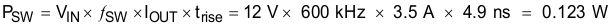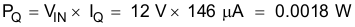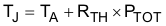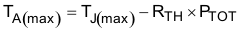JAJSBY0G August   2012  – June 2018

PRODUCTION DATA.

1. 特長
2. アプリケーション
3. 概要
1.     Device Images
4. 改訂履歴
5. Pin Configuration and Functions
6. Specifications
7. Detailed Description
1. 7.1 Overview
2. 7.2 Functional Block Diagram
3. 7.3 Feature Description
4. 7.4 Device Functional Modes
8. Application and Implementation
1. 8.1 Application Information
2. 8.2 Typical Application
1. 8.2.1 Design Requirements
2. 8.2.2 Detailed Design Procedure
3. 8.2.3 Application Curves
9. Power Supply Recommendations
10. 10Layout
1. 10.1 Layout Guidelines
2. 10.2 Layout Example
11. 11デバイスおよびドキュメントのサポート
12. 12メカニカル、パッケージ、および注文情報

• DDA|8
• DDA|8

8.2.2.13 Power Dissipation Estimate

The following formulas show how to estimate the TPS54360 power dissipation under continuous conduction mode (CCM) operation. These equations should not be used if the device is operating in discontinuous conduction mode (DCM).

The power dissipation of the IC includes conduction loss (PCOND), switching loss (PSW), gate drive loss (PGD) and supply current (PQ). Example calculations are shown with the 12 V typical input voltage of the design example.

Equation 52.spacer

Equation 53.spacer

Equation 54.spacer

Equation 55.Where:

IOUTis the output current (A).
RDS(on) is the on-resistance of the high-side MOSFET (Ω).
VOUT is the output voltage (V).
VINis the input voltage (V).
ƒswis the switching frequency (Hz).
triseis the SW terminal voltage rise time and can be estimated by trise = VIN x 0.16ns/V + 3.0ns.
QGis the total gate charge of the internal MOSFET.
IQis the operating nonswitching supply current.

Therefore,

Equation 56.For given TA,

Equation 57.For given TJMAX = 150°C

Equation 58.Where:

Ptot is the total device power dissipation (W).
TAis the ambient temperature (°C).
TJis the junction temperature (°C).
RTHis the thermal resistance of the package (°C/W).
TJMAXis maximum junction temperature (°C).
TAMAXis maximum ambient temperature (°C).

There will be additional power losses in the regulator circuit due to the inductor ac and dc losses, the catch diode and PCB trace resistance impacting the overall efficiency of the regulator.